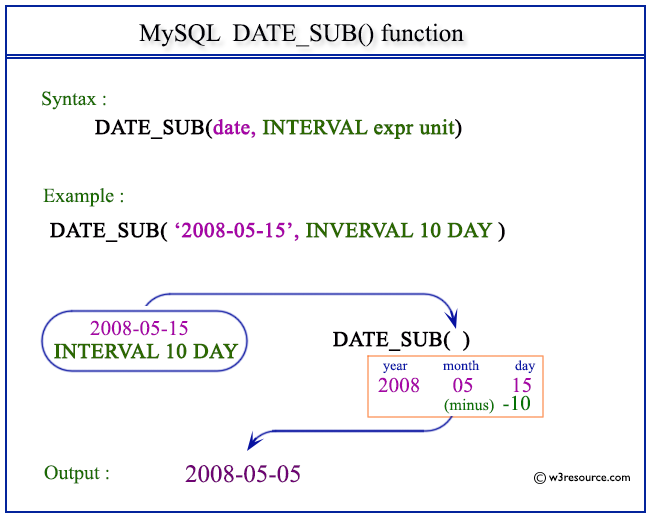# MySQL DATE_SUB() function

## DATE_SUB() function

MySQL DATE_SUB() function subtract a time value (as interval) from a date. It's a versatile function that helps perform various date and time calculations by subtracting time intervals.

This function is useful in -

• The primary purpose of the DATE_SUB() function is to perform date arithmetic by subtracting a specific interval from a given date or datetime value.
• Calculates past datetime values by subtracting time intervals, which is useful for tasks like calculating the start time of a process or tracking durations.
• The function supports a wide range of interval units, including YEAR, MONTH, DAY, HOUR, MINUTE, SECOND, etc., allowing for versatile calculations.
• DATE_SUB() is used to calculate historical dates for data analysis, allowing you to study trends and patterns over specific intervals.
• When dealing with date intervals in different units, DATE_SUB() ensures accurate conversions and calculations.
• In simulations or models that involve historical scenarios, DATE_SUB() helps set the stage by calculating past event times.

Syntax:

```DATE_SUB(date, INTERVAL expr unit)
```

Arguments:

Name Description
date A date value.
INTERVAL Keyword.
expr A date or datetime expression of a number.
unit An unit, described in the table.

MySQL Version: 8.0

Pictorial Presentation:Example: MySQL DATE_SUB() function

The following statement will return a date after subtracting 10 days from the specified date 2008-05-15.

Code:

``````SELECT DATE_SUB('2008-05-15', INTERVAL 10 DAY);
```
```

Output:

```mysql> SELECT DATE_SUB('2008-05-15', INTERVAL 10 DAY);
+-----------------------------------------+
| DATE_SUB('2008-05-15', INTERVAL 10 DAY) |
+-----------------------------------------+
| 2008-05-05                              |
+-----------------------------------------+
1 row in set (0.00 sec)
```

Example : DATE_SUB() function with 'DAY_SECOND'

The following statement will return a datetime after subtracting 1 1:10:10 DAY_SECOND from 2008-05-15 4:50:20.

Code:

``````SELECT DATE_SUB('2008-05-15 4:50:20',INTERVAL '1 1:10:10' DAY_SECOND);
```
```

Output:

```mysql> SELECT DATE_SUB('2008-05-15 4:50:20',INTERVAL '1 1:10:10' DAY_SECOND);
+----------------------------------------------------------------+
| DATE_SUB('2008-05-15 4:50:20',INTERVAL '1 1:10:10' DAY_SECOND) |
+----------------------------------------------------------------+
| 2008-05-14 03:40:10                                            |
+----------------------------------------------------------------+
1 row in set (0.00 sec)
```

Example: DATE_SUB() function with 'INTERVAL'

The following statement will return a datetime after subtracting 1 SECOND from 2008-05-15.

Code:

``````SELECT '2008-05-15' - INTERVAL 1 SECOND;
```
```

Output:

```mysql> SELECT '2008-05-15' - INTERVAL 1 SECOND;
+----------------------------------+
| '2008-05-15' - INTERVAL 1 SECOND |
+----------------------------------+
| 2008-05-14 23:59:59              |
+----------------------------------+
1 row in set (0.00 sec)
```

Example : DATE_SUB() function with plus(+) operator

The following statement will return a datetime after adding 1 HOUR with 2008-05-15. This example shows that the INTERVAL to be subtracted (or added) can also be specified before the original date / datetime.

Code:

``````SELECT INTERVAL 1 HOUR + '2008-05-15';
```
```

Output:

```mysql> SELECT INTERVAL 1 HOUR + '2008-05-15';
+--------------------------------+
| INTERVAL 1 HOUR + '2008-05-15' |
+--------------------------------+
| 2008-05-15 01:00:00            |
+--------------------------------+
1 row in set (0.00 sec)
```

Video Presentation:

All Date and Time Functions :